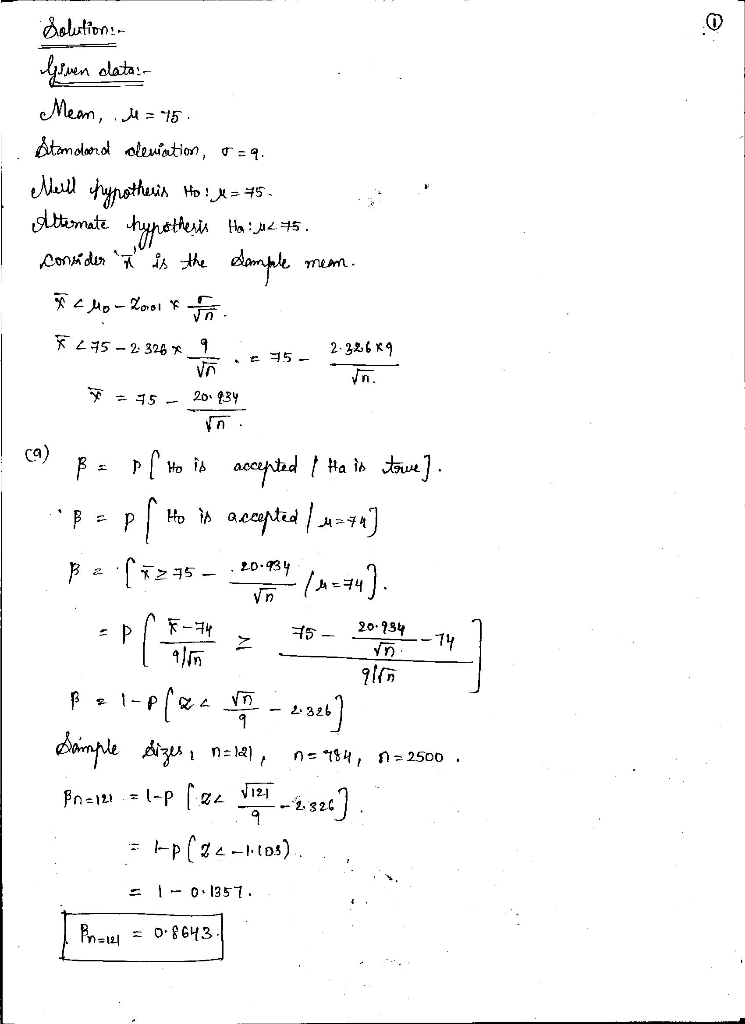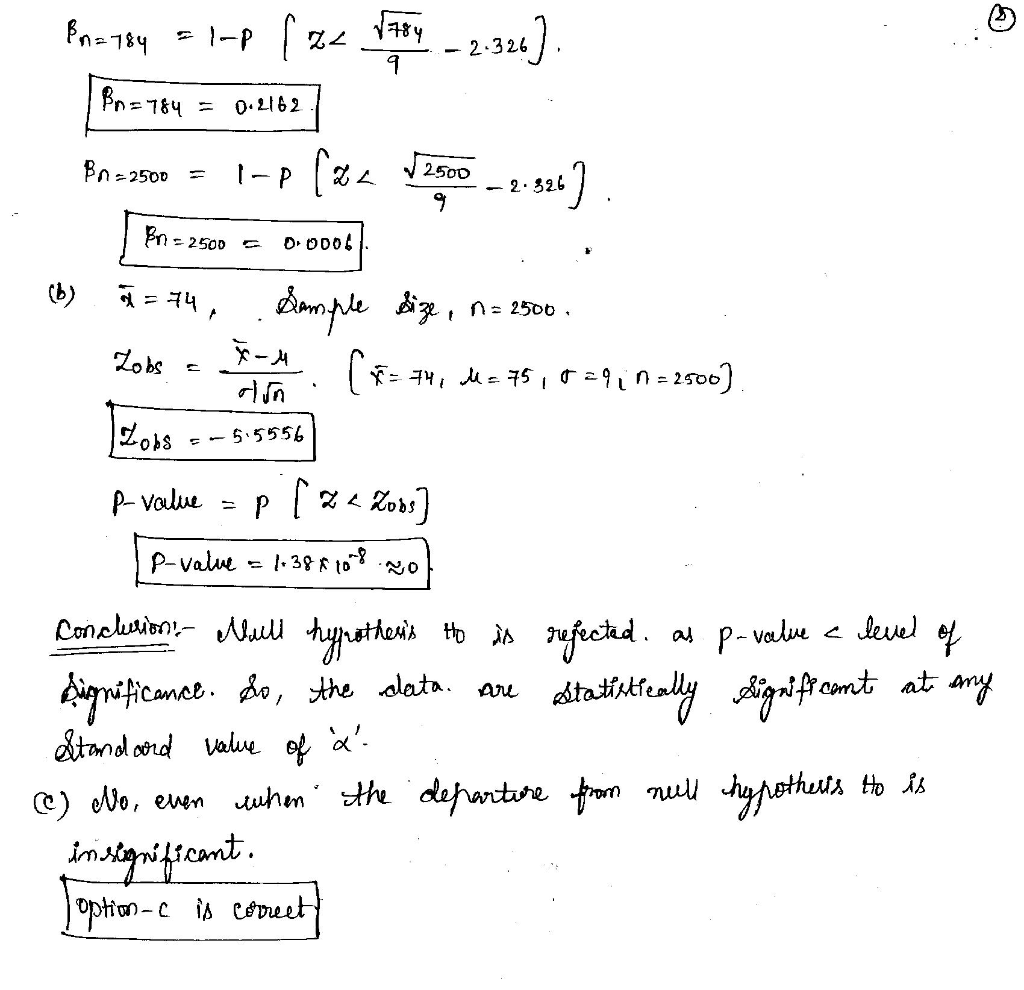Homework Help Question & Answers

The drying time of a certain type of paint under specified test conditions is known to be normall...

The drying time of a certain type of paint under specified test conditions is known to be normally distributed with mean value 75 min and standard deviation 9 min. Chemists have proposed a new additive designed to decrease average drying time. It is believed that drying times with this additive will remain normally distributed with σ = 9. Because of the expense associated with the additive, evidence should strongly suggest an improvement in average drying time before such a conclusion is adopted. Let μ denote the true average drying time when the additive is used. The appropriate hypotheses are H0: μ = 75versus Ha: μ < 75. Consider the alternative value μ = 74, which in the context of the problem would presumably not be a practically significant departure from H0.

(a) For a level 0.01 test, compute β at this alternative for sample sizes n = 121, 784, and 2500. (Round your answers to four decimal places.)

n β
121
784
2500

(b) If the observed value of

X

is

x = 74,

what can you say about the resulting P-value when n = 2500? Is the data statistically significant at any of the standard values of α? (Round your z to two decimal places. Round your P-value to four decimal places.)

 z = P-value =

State the conclusion in the problem context.

Do not reject the null hypothesis. The data are not statistically significant at any standard value of α.Reject the null hypothesis. The data are statistically significant at any standard value of α.    Reject the null hypothesis. The data are not statistically significant at any standard value of α.Do not reject the null hypothesis. The data are statistically significant at any standard value of α.

(c) Would you really want to use a sample size of 2500 along with a level 0.01 test (disregarding the cost of such an experiment)? Explain.

Yes, even when the departure from H0 is significant from a practical point of view, a statistically significant result is not likely to appear; it is difficult for the test to detect departures from H0.Yes, it is always advantageous to have a very large sample size, because it will detect very small departures from H0.    No, even when the departure from H0 is insignificant from a practical point of view, a statistically significant result is highly likely to appear; the test is too likely to detect small departures from H0.No, it is never advantageous to have a very large sample size, because it cannot detect very small departures from H0.

You may need to use the appropriate table in the Appendix of Tables to answer this question.Add Answer of: The drying time of a certain type of paint under specified test conditions is known to be normall...
More Homework Help Questions Additional questions in this topic.

• 2. The followving data give the drying time T of a certain pauin n t a certain additive A. Find the first, second, third, and fourth-degree polynomials that fit the data and plot each polynomial...

Need Online Homework Help?

Get FREE EXPERT Answers
WITHIN MINUTES
Related Questions